# High School Physics : Electric Charge

## Example Questions

### Example Question #921 : High School Physics

Materials in which the electrons are bound very loosely to the nuclei and can move about freely within the material are referred to as

Semiconductors

Conductors

Superconductors

Insulators

Conductors

Explanation:

Conductors allow the electrons to flow freely along it.  That is why metal; is considered a good conductor.  It allows the electrons to flow through it which is why it is used in wire in an an electric circuit.

### Example Question #1 : Electric Charge

A glass rod is rubbed with a piece of silk.  During the process the glass rod acquires a positive charge.  The silk

Could either be positively charged or negatively charged.  It depends on how hard the rod was rubbed.

Remains neutral

Acquires a positive charge as well

Acquires a negative charge

Acquires a negative charge

Explanation:

Since the glass rod acquires a positive charge, this means that it is deficient in electrons. Since the rod was rubbed by the piece of silk, the silk is what now collects the electrons.  The silk now has an excess of electrons which means that the silk is now negatively charged.

### Example Question #1 : Electric Charge

A charged rod carrying a negative charge is brought near two spheres that are in contact with each other but insulated from the ground. If the two spheres are then separated, what kind of charge will be on the spheres?

None of these

The sphere near the charged rod becomes positive and the other becomes negative

The spheres do not get any charge

The sphere near the charged rod becomes negative and the other becomes positive

The sphere near the charged rod becomes positive and the other becomes negative

Explanation:

When the negatively charged rod is brought near one of the two spheres, the presents of the negative charge will induce a flow of charge in the spheres such that regions farthest away from the charged rod will become most negative and regions near the rod will become most positive. This is called charge by induction.

### Example Question #921 : High School Physics

By what method will a positively charged rod produce a negative charge on a conducting sphere that is placed on an insulating surface?

Charge by conduction

Charge by convection

None of these

Charge by induction

Charge by induction

Explanation:

Charge by induction happens when a charged object is brought in the vicinity of a neutral object. The presents of the charged object will cause the free charges in the neutral object to shift such that the neutral object becomes polarized. When the charged object is positive, this will induce a negative charge on a neutral object.

### Example Question #925 : High School Physics

How many electrons make up a charge of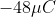?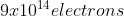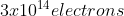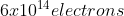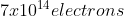Explanation:

The charge of a single electron is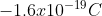We can then convert the amount of charge to determine the number of electrons.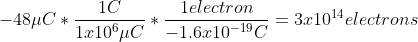### Example Question #926 : High School Physics

A neutral atom always has

The same number of protons and electrons

More neutrons than protons

The same number of neutrons and protons

More protons than electrons

The same number of protons and electrons

Explanation:

Protons are positively charged and electrons are negatively charged.  In order for an object to be considered neutral, it must have the same number of both positive and negative particles.  Therefore it must have the same number of both protons and electrons.

### Example Question #71 : Electricity And Magnetism

As an object acquires a positive charge, its mass usually

Becomes negative

Increases

Decreases

Stays the same

Decreases

Explanation:

When an object acquires a positive charge, it is losing electrons to its surroundings.  If it is losing electrons, it is losing mass (although ever so slightly).

### Example Question #928 : High School Physics

What is the net charge of abar of gold?  Gold has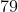electrons per atom and an atomic mass of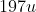.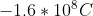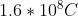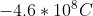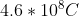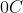Explanation:

Since a gold atom has balanced protons and electrons, the net charge on the atom is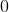.

### Example Question #1 : Electric Charge

A negative point charge is in an electric field created by a positive point charge. Which of the following is true?

The field points away from the positive charge, and the force on the negative charge is in the same direction as the field

The field points toward the positive charge and the force on the negative charge is in the same direction as the field

The field points toward the positive charge and the force on the negative charge is in the opposite direction as the field

The field points away from the positive charge, and the force on the negative charge is in the opposite direction as the field

The field points away from the positive charge, and the force on the negative charge is in the opposite direction as the field

Explanation:

By convention, electric fields point away from positive charges and toward negative charges. Since the field is created by a positive point charge, the electric field will point away from the positive charge.

Negative and positive charges attract one another. Therefore the force on the negative charge is toward the positive charge. This is in the opposite direction as the field.

### Example Question #921 : High School Physics

When an object such as a plastic comb is charged by rabbit it with a cloth, the net charge is typically a few microcoulombs. If that charge is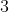, by what amount does the mass of a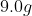comb change during charging?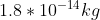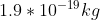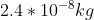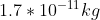Explanation:

First, we need to determine how many additional electrons are on the comb due to the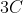of charge.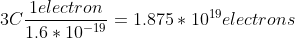Now that we know the number of electrons, we can determine the mass of these additional electrons.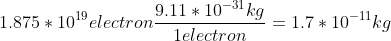### All High School Physics Resources